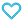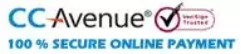# MTG 100 Percent Mathematics Class-9 Term 1 and Term 2

575.00

Discount offer on this book in a bundle, click to view

‘MTG 100 PERCENT Maths’ aims to provide students with a thorough understanding of mathematic concepts and provide 100 percent learning. The book is updated with the latest revised syllabus (2021-22) useful for Term 1 & 2 accompanying with comprehensive theory, illustrations, graphics, concept maps. Also supplemented with a series of MCQs, VSA, SA, and LA, Assertion & Reason, Numerical Value type questions in the sections of Try Yourself, NCERT focus, CBSE Focus, Competition Focus and Exam drill with detailed solutions. Term Tests are given to prepare students for internal assessments. 3 Practice papers (solved) as per the latest design and blue print of CBSE for the current academic session are provided.

949 in stock

Qty:Add to Wishlist100 percent as the name suggest is a mission to score 100% in your CBSE exams. The motive behind curating this title ‘MTG 100 PERCENT Mathematics’ is to render students a deep insight into Mathematics at secondary level and help them conquer their fears and score brilliant in the exam by achieving all learning objectives. The content of this student friendly book will hit all the three domains of learning viz. cognitive, affective and psychomotor.

Focussed on covering all the elements that a CBSE students urge for, this book comprises of:

• Unparalleled Theory: Theory of Mathematical concepts is presented in a elaborated yet in an interesting way so that students won’t lose interest in the subject. To build practical skills of the students, a number of activities have been introduced in each chapter in the name of ‘Lab Time’.
• Illustrations and Evaluation Columns: Each section of theory is followed by self-test assignments in the form of Try Yourself. NCERT Focus and CBSE Focus exercises are given to make the students familiar with the types of NCERT Textbook and CBSE Board questions.
• Concept Map – The Recapitulation Tool: A well designed concept map is provided at the end of theory of each chapter that summarises the main points of the chapter.
• Chapter Exercise: Chapter exercise covers all possible type and pattern of questions. Each chapterwise paper is designed as per the CBSE pattern in the section “exam drill”.
• Term Test: Pre-Mid Term, Mid Term and Post-Mid Term tests are given to prepare the students for internal assessments.
• Practice Papers: 3 Practice papers (solved) as per the latest design and blue print of CBSE for the current academic session along with their complete solutions are provided.

In true sense this is a kind of book that will definitely help you aiming 100% in your CBSE Exams.

Table of Content:-
• Chapter 1. Number Systems
• Chapter 2. Polynomials
• Chapter 3. Coordinate Geometry
• Chapter 4. Linear Equations in Two Variables
• Chapter 5. Introduction to Euclid's Geometry
• Chapter 6. Lines and Angles
• Chapter 7. Triangles
• Chapter 9. Areas of Parallelograms and Triangles
• Chapter 10. Circles
• Chapter 11. Constructions
• Chapter 12. Heron's Formula
• Chapter 13. Surface Areas and Volumes
• Chapter 14. Statistics
• Chapter  15. Probability
• Post Mid Term
• 3 Practice Papers
ISBN11 9789390931804 MTG Editorial Board 2021-22 542 Class 9 School Books Mathematics 928gm

## Reviews

There are no reviews yet.